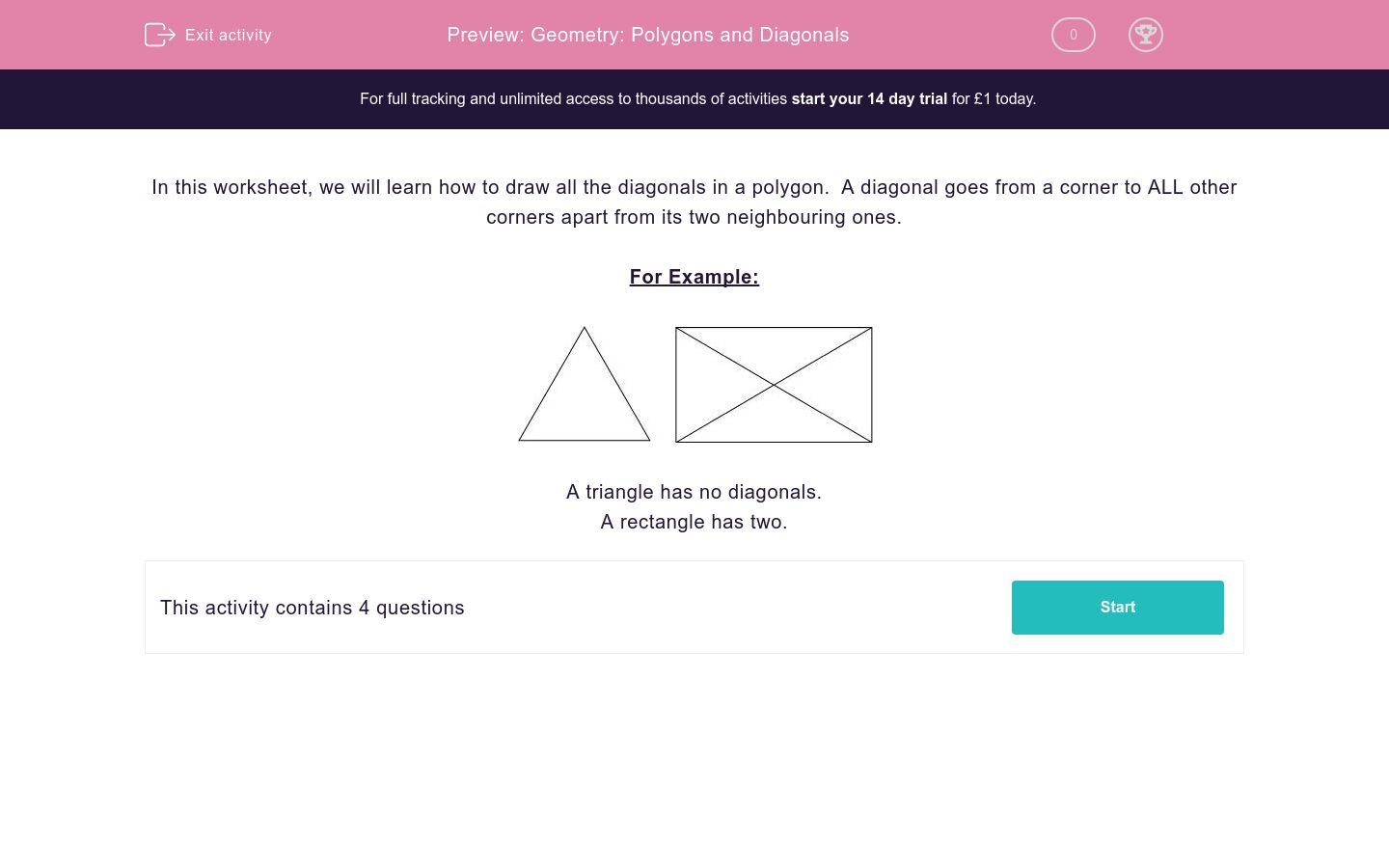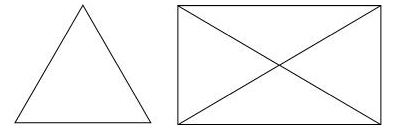# Geometry: Polygons and Diagonals

In this worksheet, students learn about diagonals in polygons.Key stage:  KS 2

Curriculum topic:   Maths and Numerical Reasoning

Curriculum subtopic:   2D Shapes: Triangles, Quadrilaterals and Polygons

Difficulty level:### QUESTION 1 of 10

In this worksheet, we will learn how to draw all the diagonals in a polygon.  A diagonal goes from a corner to ALL other corners apart from its two neighbouring ones.

For Example:A triangle has no diagonals.

A rectangle has two.

---- OR ----